# Solving Partial Differential Equations with Octave

## PDEONE + the Runge Kutta Chebyshev ODE integrator rkc.f

This is the first release of some code I have written for solving one-dimensional partial differential equations with Octave. The types of equations that can be solved with this method are of the following form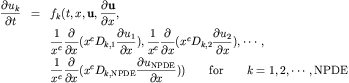Here the vector u is a vector of unknowns that depends on both space and time. The spatial domain x is finite i.e. [a,b]. Here u_x is the first partial derivative of the vector of unknowns and the second derivative information is specified by specifying the "diffusion" functions D. The diffusion coefficients "D" can be functions of x,t, and u. In addition, the constant c above can be 0, 1, or 2 depending on whether the problem is specified in Cartesian, cylindrical, or spherical coordinates. Boundary conditions depend on the partial differential equation (PDE) solved and are imposed in the octave code as equations of the following form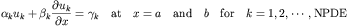Here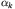,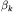, and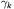are user defined vector valued functions which may depend on t and u if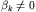but only functions of x otherwise. The initial conditions are specified for each component of u at the initial time t_0. These initial conditions don't necessarily have to be consistent with the boundary conditions above.
To solve PDE's with PDEONE in octave the user must specify octave functions (with a very similar format to Matlab functions) defining each of the functions described above for the specific problem at hand. As such it is very easy to modify and solve various different PDES. One can use the same functional call and just change the octave functions references. Various examples of this use are provided in the software below. The code is currently a C++/octave API wrapper that calls the core solution routine PDEONE described in the paper:

Algorithm 494: PDEONE, Solutions of Systems of Partial Differential Equations
by R. F. Sincovec and N. K. Madsen
ACM Transactions on Mathematical Software (TOMS), Volume 1 Issue 3 (September 1975)

As a check for errors I have be able to duplicate all of the non-stiff results from that paper using the code provided here (see below). Next one will find a subset of some of the examples provided there. Note: all examples from that paper are provided in this software delivery and work as well as the included ODE solver can be expected to (see below).

## Examples:

Note: that the reaction-diffusion example (above) is not from the Sincovec and Madsen paper but was provided with the rkc ODE solver as an example of is application in the method of lines. I should mention that this version of PDEONE uses the numerical ODE integrator "rkc.f" provided by Sommeijer, Shampine, and Verwer which can be found on netlib. The ODE solver used in the original Sincovec and Madsen paper was Hindmarsh's integrator GEARB (see references in the paper above). At the time of this programs development I couldn't find an electronic version of this code and so used a Runge Kutta Chebyshev ODE solver instead. Some comments about this solver are in order. The rkc solver is an explicit method with very low memory requirements and a quadratically growing stability region. As such it is able to dynamically select, at each step, the most efficient stable formula. These properties make it ideal for the solution of parabolic partial differential equations using the method of lines. In fact it is presented explicitly in such a light in the paper below. The drawback in using such a solver is that when the partial differential equation to be solved is stiff (more hyperbolic than parabolic) this solver can fail. When attempting to duplicate the results of the Sincovec and Madsen paper on several of the examples this happened. Practically, this means that the rkc solver takes unbearable a long time while it iterates looking for an acceptable stability region. This could be monitored by considering the output of the variable MAXM but is currently not implemented yet. It should be mentioned that as a quick fix often one can refine the grid or extend the domain and the solver will be able to proceed. A more permanent solution is to implement a stiff ODE solver (such as the GEARB ODE solver). In the results presented here the rkc solver performed as well as could be expected (which was quite well). The Sommeijer, Shampine, and Verwer ODE solver "rkc" is described in more detail in the following reference:

B.P. Sommeijer, L.F. Shampine and J.G. Verwer
RKC: an Explicit Solver for Parabolic PDEs.
Technical Report MAS-R9715, CWI, Amsterdam, 1997

The license in the ACM code only allows non-commercial usage of their codes. This license is too restrictive for the Free Software Foundation GNU copyleft and therefore the code cannot be included in the mainstream Octave sources. This license does however permit usage of this software for educational purposes.

Please reference this software in any publications that result from its use. A sample bibtex entry is below

``` @misc{weatherwaxPDEONEG, author = "J L. Weatherwax", title = "Software for solving PDE's with Octave", text = "PDEONEG: An Octave Gateway Routine to pdeone.f", year = "2005", url = "http://web.mit.edu/wax/www/Software/pdeone.html" } ```

I should mention that I am very interested in having people use this software and as such would be very willing to help get people started using it. As always, please send any comments to the address below.

## Code Versions:

• The version 1.1 now allows the user to enter the initial conditions as a matrix in addition to being able to specify them as an octave function. This allows the user to use this code to perform fractional splitting like techniques on their equations. By this I mean one could decompose the initial problem into two subproblems and solve the total problem by solving a sequence of the two subproblems. In this sequence of subproblems the solution to the firs problem is used as the initial condition to the next problem in sequence and one must be able to specify initial conditions as a matrix. There is a nice discussion of this technique in Chapter 17 in Randall LeVeque's book "Finite Volume Methods For Hyperbolic Problems".
• The version 1.0 has all the examples from the Sincovec and Madsen paper working and implemented, plus some additional error checking.
• The version 0.5 code has had a few bugs fixed from the 0.2 release and many more examples from the Sincovec and Madsen paper implemented.
• The version 0.2 code works nicely and is able to duplicate the results from the first example given in the paper references above. See the example.

## Installation

### General Notes:

The code was compiled on a 686 athalon chip and thus should not need to be recompiled if you are using a similar chipset. If this is not the case to rebuild the function you will need a FORTRAN 77 compiler and a C++ compiler in addition to the mkoctfile script to produce the dynamically linked function pdeoneg.oct.

### Linux/Unix:

To run all the examples provided you first must extract the source into a local directory. In this example, lets assume that you downloaded a file named "PDEONE.x.y". Where x and y are the major and minor version numbers PDEONE distribution. First unzip and untar the distribution using

``` gunzip PDEONE.x.y.tar.gz tar -xvf filename.tar ```

Where `filename` is the name of the version of code downloaded. Next, change into the newly created directory

``` cd PDEONE.x.y ```

In that directory, one should see subdirectories containing the various octave files used to specify the different PDE's. The first numerical PDE example from the paper above is in the subdirectory is named "EG1". To run the code corresponding to this PDE definition. We must first insure that octave can find these files and not any others with the same name. As such there should be a file called `.octaverc` in this top most directory. Open this file in an editor and change the line to read (if it is not already)

``` LOADPATH = ['EG1//', LOADPATH]; ```

Now start octave in this top most directory, with an invocation like ``` octave & ```

The `LOADPATH` command will set the path so that when octave is started in the given directory it will find the required `pdeone_Script.m` in the EG1 directory and no other. Then at the octave prompt type

``` pdeone_Script ```

and the given PDE will be solved numerically for you with a few plots produced.

### Windows:

I developed the PDEONE package on a Linux system and have never tested it on Windows, but it should work with if the proper compilers are provided and you remake the dynamically liked function pdeoneg.oct. See the discussion above. If you are able to get this to work I would be happy to hear about it including tricks you had to know or perform to get everything to work.

## To Do:

• Replace the ODE solver RKC with Hindmarsh's GEARB integrator which (I now know) can be found with the PDETWO source code or another implicit ODE solver.
• Make the passage of arguments to all of the user defined functions easy.
• Include more examples of solved PDE's using this code on the web.
• More complete and better documentation.